# How to Sort a Pandas DataFrame based on column names or row index?

Pandas` dataframe.sort_index()` method sorts objects by labels along the given axis. Basically the sorting algorithm is applied on the axis labels rather than the actual data in the dataframe and based on that the data is rearranged. We have the freedom to choose what sorting algorithm we would like to apply. There are three possible sorting algorithms that we can use ‘quicksort’, ‘mergesort’, and ‘heapsort’.

Syntax: DataFrame.sort_index(axis=0, level=None, ascending=True, inplace=False, kind=’quicksort’, na_position=’last’, sort_remaining=True, by=None)

Parameters :
axis : p for index, 1 for columns to direct sorting
level : if not None, sort on values in specified index level(s)
ascending : Sort ascending vs. descending
inplace : if True, perform operation in-place i.e changes in actual dataframe otherwise return a sorted dataframe.
kind : {‘quicksort’, ‘mergesort’, ‘heapsort’}, default ‘quicksort’. Choice of sorting algorithm. See also ndarray.np.sort for more information. mergesort is the only stable algorithm. For DataFrames, this option is only applied when sorting on a single column or label.
na_position : [{‘first’, ‘last’}, default ‘last’] First puts NaNs at the beginning, last puts NaNs at the end. Not implemented for MultiIndex.
sort_remaining : If true and sorting by level and index is multilevel, sort by other levels too (in order) after sorting by specified level

Return : sorted_obj : DataFrame

Create a simple dataframe with a dictionary of lists, say column names are: ‘Name’, ‘Age’, ‘Place’ and ‘College’.

 `# import pandas library as pd ` `import` `pandas as pd ` ` `  `# List of Tuples ` `students ``=` `[(``'Ankit'``, ``22``, ``'Up'``, ``'Geu'``), ` `           ``(``'Ankita'``, ``31``, ``'Delhi'``, ``'Gehu'``), ` `           ``(``'Rahul'``, ``16``, ``'Tokyo'``, ``'Abes'``), ` `           ``(``'Simran'``, ``41``, ``'Delhi'``, ``'Gehu'``), ` `           ``(``'Shaurya'``, ``33``, ``'Delhi'``, ``'Geu'``), ` `           ``(``'Harshita'``, ``35``, ``'Mumbai'``, ``'Bhu'` `), ` `           ``(``'Swapnil'``, ``35``, ``'Mp'``, ``'Geu'``), ` `           ``(``'Priya'``, ``35``, ``'Uk'``, ``'Geu'``), ` `           ``(``'Jeet'``, ``35``, ``'Guj'``, ``'Gehu'``), ` `           ``(``'Ananya'``, ``35``, ``'Up'``, ``'Bhu'``) ` `            ``] ` ` `  `# Create a DataFrame object from ` `# list of tuples with columns ` `# and indices. ` `details ``=` `pd.DataFrame(students, columns ``=``[``'Name'``, ``'Age'``, ` `                                           ``'Place'``, ``'College'``], ` `                        ``index ``=``[ ``'b'``, ``'c'``, ``'a'``, ``'e'``, ``'f'``, ``'g'``, ` `                                 ``'i'``, ``'j'``, ``'k'``, ``'d'``]) ` `# show the dataframe ` `details `

Output: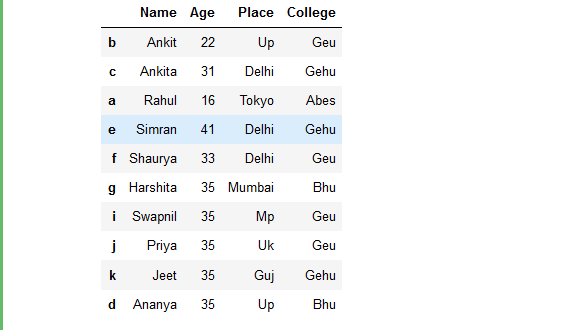Example 1: Sort the rows of dataframe based on row index label names.

 `# import pandas library as pd ` `import` `pandas as pd ` ` `  `# List of Tuples ` `students ``=` `[(``'Ankit'``, ``22``, ``'Up'``, ``'Geu'``), ` `           ``(``'Ankita'``, ``31``, ``'Delhi'``, ``'Gehu'``), ` `           ``(``'Rahul'``, ``16``, ``'Tokyo'``, ``'Abes'``), ` `           ``(``'Simran'``, ``41``, ``'Delhi'``, ``'Gehu'``), ` `           ``(``'Shaurya'``, ``33``, ``'Delhi'``, ``'Geu'``), ` `           ``(``'Harshita'``, ``35``, ``'Mumbai'``, ``'Bhu'` `), ` `           ``(``'Swapnil'``, ``35``, ``'Mp'``, ``'Geu'``), ` `           ``(``'Priya'``, ``35``, ``'Uk'``, ``'Geu'``), ` `           ``(``'Jeet'``, ``35``, ``'Guj'``, ``'Gehu'``), ` `           ``(``'Ananya'``, ``35``, ``'Up'``, ``'Bhu'``) ` `            ``] ` ` `  `# Create a DataFrame object from ` `# list of tuples with columns ` `# and indices. ` `details ``=` `pd.DataFrame(students, columns ``=``[``'Name'``, ``'Age'``,  ` `                                           ``'Place'``, ``'College'``], ` `                        ``index ``=``[ ``'b'``, ``'c'``, ``'a'``, ``'e'``, ``'f'``,  ` `                                ``'g'``, ``'i'``, ``'j'``, ``'k'``, ``'d'``]) ` ` `  `# sort the rows of dataframe  ` `# based on row index ` `rslt_df ``=` `details.sort_index() ` ` `  `# show the resultant Dataframe ` `rslt_df `

Output: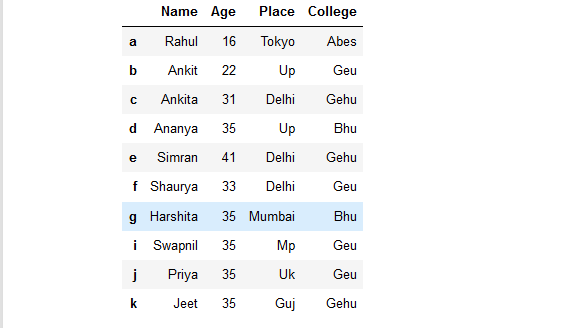Example 2: Sort rows of a Dataframe in Descending Order based on Row index labels.

 `# import pandas library as pd ` `import` `pandas as pd ` ` `  `# List of Tuples ` `students ``=` `[(``'Ankit'``, ``22``, ``'Up'``, ``'Geu'``), ` `           ``(``'Ankita'``, ``31``, ``'Delhi'``, ``'Gehu'``), ` `           ``(``'Rahul'``, ``16``, ``'Tokyo'``, ``'Abes'``), ` `           ``(``'Simran'``, ``41``, ``'Delhi'``, ``'Gehu'``), ` `           ``(``'Shaurya'``, ``33``, ``'Delhi'``, ``'Geu'``), ` `           ``(``'Harshita'``, ``35``, ``'Mumbai'``, ``'Bhu'` `), ` `           ``(``'Swapnil'``, ``35``, ``'Mp'``, ``'Geu'``), ` `           ``(``'Priya'``, ``35``, ``'Uk'``, ``'Geu'``), ` `           ``(``'Jeet'``, ``35``, ``'Guj'``, ``'Gehu'``), ` `           ``(``'Ananya'``, ``35``, ``'Up'``, ``'Bhu'``) ` `            ``] ` ` `  `# Create a DataFrame object from ` `# list of tuples with columns ` `# and indices. ` `details ``=` `pd.DataFrame(students, columns ``=``[``'Name'``, ``'Age'``, ` `                                           ``'Place'``, ``'College'``], ` `                        ``index ``=``[ ``'b'``, ``'c'``, ``'a'``, ``'e'``, ``'f'``,  ` `                                ``'g'``, ``'i'``, ``'j'``, ``'k'``, ``'d'``]) ` ` `  `# sort the rows of dataframe in descending  ` `# order based on row index  ` `rslt_df ``=` `details.sort_index(ascending ``=` `False``) ` ` `  `# show the resultant Dataframe ` `rslt_df `

Output: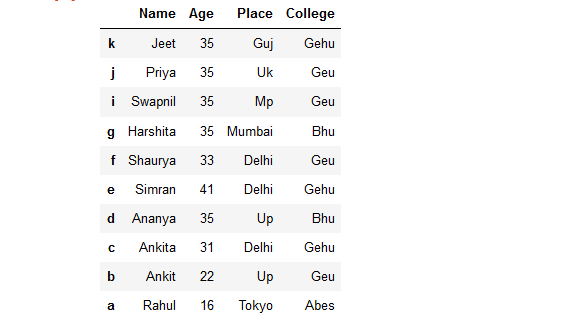Example 3: Sort rows of a Dataframe based on Row index labels in Place.

 `# import pandas library as pd ` `import` `pandas as pd ` ` `  `# List of Tuples ` `students ``=` `[(``'Ankit'``, ``22``, ``'Up'``, ``'Geu'``), ` `           ``(``'Ankita'``, ``31``, ``'Delhi'``, ``'Gehu'``), ` `           ``(``'Rahul'``, ``16``, ``'Tokyo'``, ``'Abes'``), ` `           ``(``'Simran'``, ``41``, ``'Delhi'``, ``'Gehu'``), ` `           ``(``'Shaurya'``, ``33``, ``'Delhi'``, ``'Geu'``), ` `           ``(``'Harshita'``, ``35``, ``'Mumbai'``, ``'Bhu'` `), ` `           ``(``'Swapnil'``, ``35``, ``'Mp'``, ``'Geu'``), ` `           ``(``'Priya'``, ``35``, ``'Uk'``, ``'Geu'``), ` `           ``(``'Jeet'``, ``35``, ``'Guj'``, ``'Gehu'``), ` `           ``(``'Ananya'``, ``35``, ``'Up'``, ``'Bhu'``) ` `            ``] ` ` `  `# Create a DataFrame object from ` `# list of tuples with columns ` `# and indices. ` `details ``=` `pd.DataFrame(students, columns ``=``[``'Name'``, ``'Age'``, ` `                                           ``'Place'``, ``'College'``], ` `                        ``index ``=``[ ``'b'``, ``'c'``, ``'a'``, ``'e'``, ``'f'``,  ` `                                ``'g'``, ``'i'``, ``'j'``, ``'k'``, ``'d'``]) ` ` `  `# sort the rows of dataframe in Place  ` `# based on row index  ` `details.sort_index(inplace ``=` `True``) ` ` `  `# show the resultant Dataframe ` `details `

Output: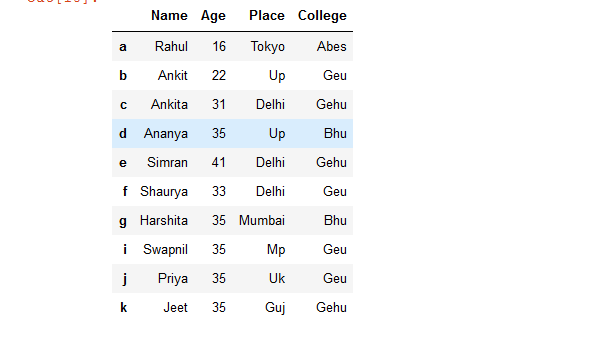Example 4: Sort Columns of a Dataframe based on Column Names.

 `# import pandas library as pd ` `import` `pandas as pd ` ` `  `# List of Tuples ` `students ``=` `[(``'Ankit'``, ``22``, ``'Up'``, ``'Geu'``), ` `           ``(``'Ankita'``, ``31``, ``'Delhi'``, ``'Gehu'``), ` `           ``(``'Rahul'``, ``16``, ``'Tokyo'``, ``'Abes'``), ` `           ``(``'Simran'``, ``41``, ``'Delhi'``, ``'Gehu'``), ` `           ``(``'Shaurya'``, ``33``, ``'Delhi'``, ``'Geu'``), ` `           ``(``'Harshita'``, ``35``, ``'Mumbai'``, ``'Bhu'` `), ` `           ``(``'Swapnil'``, ``35``, ``'Mp'``, ``'Geu'``), ` `           ``(``'Priya'``, ``35``, ``'Uk'``, ``'Geu'``), ` `           ``(``'Jeet'``, ``35``, ``'Guj'``, ``'Gehu'``), ` `           ``(``'Ananya'``, ``35``, ``'Up'``, ``'Bhu'``) ` `            ``] ` ` `  `# Create a DataFrame object from ` `# list of tuples with columns ` `# and indices. ` `details ``=` `pd.DataFrame(students, columns ``=``[``'Name'``, ``'Age'``,  ` `                                           ``'Place'``, ``'College'``], ` `                        ``index ``=``[ ``'b'``, ``'c'``, ``'a'``, ``'e'``, ``'f'``,  ` `                                ``'g'``, ``'i'``, ``'j'``, ``'k'``, ``'d'``]) ` ` `  `# sort a dataframe based on column names ` `rslt_df ``=` `details.sort_index(axis ``=` `1``) ` ` `  `# show the resultant Dataframe ` `rslt_df `

Output: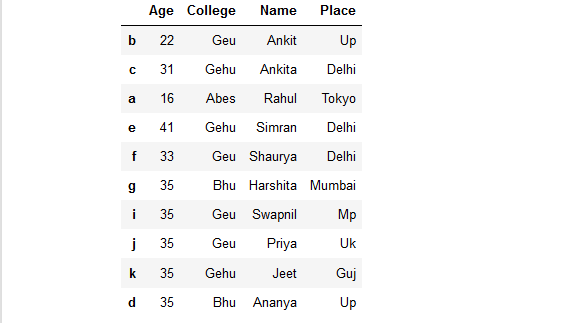Example 5: Sort a dataframe in descending order based on column names.

 `# import pandas library as pd ` `import` `pandas as pd ` ` `  `# List of Tuples ` `students ``=` `[(``'Ankit'``, ``22``, ``'Up'``, ``'Geu'``), ` `           ``(``'Ankita'``, ``31``, ``'Delhi'``, ``'Gehu'``), ` `           ``(``'Rahul'``, ``16``, ``'Tokyo'``, ``'Abes'``), ` `           ``(``'Simran'``, ``41``, ``'Delhi'``, ``'Gehu'``), ` `           ``(``'Shaurya'``, ``33``, ``'Delhi'``, ``'Geu'``), ` `           ``(``'Harshita'``, ``35``, ``'Mumbai'``, ``'Bhu'` `), ` `           ``(``'Swapnil'``, ``35``, ``'Mp'``, ``'Geu'``), ` `           ``(``'Priya'``, ``35``, ``'Uk'``, ``'Geu'``), ` `           ``(``'Jeet'``, ``35``, ``'Guj'``, ``'Gehu'``), ` `           ``(``'Ananya'``, ``35``, ``'Up'``, ``'Bhu'``) ` `            ``] ` ` `  `# Create a DataFrame object from ` `# list of tuples with columns ` `# and indices. ` `details ``=` `pd.DataFrame(students, columns ``=``[``'Name'``, ``'Age'``,  ` `                                           ``'Place'``, ``'College'``], ` `                        ``index ``=``[ ``'b'``, ``'c'``, ``'a'``, ``'e'``, ``'f'``,  ` `                                ``'g'``, ``'i'``, ``'j'``, ``'k'``, ``'d'``]) ` ` `  `# sort a dataframe in descending  ` `# order based on column names ` `rslt_df ``=` `details.sort_index(ascending ``=` `False``, axis ``=` `1``) ` ` `  `# show the resultant Dataframe ` `rslt_df `

Output: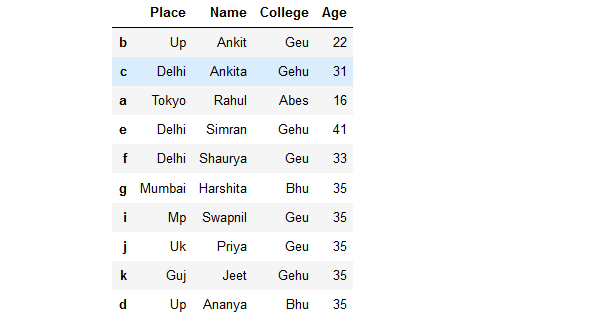Example 6: Sort a dataframe in place based on column names.

 `# import pandas library as pd ` `import` `pandas as pd ` ` `  `# List of Tuples ` `students ``=` `[(``'Ankit'``, ``22``, ``'Up'``, ``'Geu'``), ` `           ``(``'Ankita'``, ``31``, ``'Delhi'``, ``'Gehu'``), ` `           ``(``'Rahul'``, ``16``, ``'Tokyo'``, ``'Abes'``), ` `           ``(``'Simran'``, ``41``, ``'Delhi'``, ``'Gehu'``), ` `           ``(``'Shaurya'``, ``33``, ``'Delhi'``, ``'Geu'``), ` `           ``(``'Harshita'``, ``35``, ``'Mumbai'``, ``'Bhu'` `), ` `           ``(``'Swapnil'``, ``35``, ``'Mp'``, ``'Geu'``), ` `           ``(``'Priya'``, ``35``, ``'Uk'``, ``'Geu'``), ` `           ``(``'Jeet'``, ``35``, ``'Guj'``, ``'Gehu'``), ` `           ``(``'Ananya'``, ``35``, ``'Up'``, ``'Bhu'``) ` `            ``] ` ` `  `# Create a DataFrame object from ` `# list of tuples with columns ` `# and indices. ` `details ``=` `pd.DataFrame(students, columns ``=``[``'Name'``, ``'Age'``,  ` `                                           ``'Place'``, ``'College'``], ` `                        ``index ``=``[ ``'b'``, ``'c'``, ``'a'``, ``'e'``, ``'f'``,  ` `                                ``'g'``, ``'i'``, ``'j'``, ``'k'``, ``'d'``]) ` ` `  `# sort a dataframe in place  ` `# based on column names ` `details.sort_index(inplace ``=` `True``, axis ``=` `1``) ` ` `  `# show the resultant Dataframe ` `details `

Output: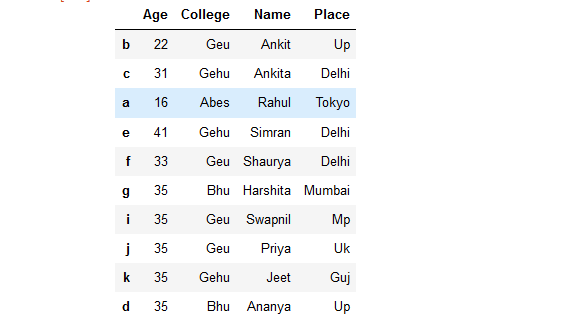Attention geek! Strengthen your foundations with the Python Programming Foundation Course and learn the basics.

To begin with, your interview preparations Enhance your Data Structures concepts with the Python DS Course.

My Personal Notes arrow_drop_upCheck out this Author's contributed articles.

If you like GeeksforGeeks and would like to contribute, you can also write an article using contribute.geeksforgeeks.org or mail your article to contribute@geeksforgeeks.org. See your article appearing on the GeeksforGeeks main page and help other Geeks.

Please Improve this article if you find anything incorrect by clicking on the "Improve Article" button below.

Article Tags :

Be the First to upvote.

Please write to us at contribute@geeksforgeeks.org to report any issue with the above content.